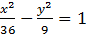### Sample Problem

Given that, what are the vertices, foci, and asymptotes of this hyperbola? If an answer is not a whole number, leave it in decimal form rounded to the nearest tenth.

Vertices: ( ± , 0)

Foci: ( ± , 0)

Asymptotes: y = ± x

#### Solution

The vertices are located at ( ±a, 0); since a = 6, the vertices are (±6,0)

c2=a2+b2 = 36 + 9 = 45

c =c ≈ 6.7, so the foci are located at ( ± 6.7 , 0)

The asymptotes are y =, so y =±; y = ±0.5x